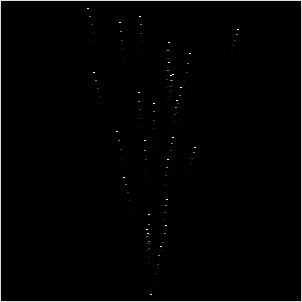## How to make fireworks, fountains, etc in processing

Particle effects look very fancy but are actually very easy to implement in processing. This is a small tutoral that shows how. The particle effect implemented here is 2-Dimentional but the same principles can be applied to a 3D version.The first thing we need are arrays to store the position and the velocity of the particles we are creating and the number of particles we want to create.

``````int NUM = 40;
PVector[] pos;
PVector[] vel;
``````

we also need an array to store the age of a particle to make it dissapear after some time (fireworks particle that fade, water that drops back in the fountain, fume particles that slide up into the air, ...)

``````int[] ttl;
``````

Now comes the usual setup method where we initialize the size of our sketch-window and fill our arrays.

``````void setup() {
size(300,300);
frameRate(25);

pos = new PVector[NUM];
vel = new PVector[NUM];
ttl = new int[NUM];
for( int i = 0; i < NUM; i++) {
pos[i] = new PVector(0,0);
if (i < NUM/2) {
vel[i] = new PVector(random(-2,2), random(-10,-5));
ttl[i] = int(random(50,100));
} else {
vel[i] = new PVector(0,0);
ttl[i] = int(random(0,50));
}
}
}
``````

We place all particles at the origin and make them go upward. For the first halve of the particles we define a velocity that points upward, the rest stays at the origin for now. we also set the ttl value for every particle to a random value between 50 and 100. We do this to prevent all particles we define to move away from the origin in an big burst at the start of our sketch.

next thing we need is our draw method. Here we will draw our particle and update the position. We also decrease the ttl value for every particle. If the ttl falls below zero we recreate the particle at the origin and assign it a new velocity and ttl. To update the position of the current particle we simply add it's velocity. Maybe you vagually remember the s = s0 + t * v stuff your math- or physics-Teacher tried you to remember - this is exactly what we are doing here)

``````void draw() {
background(0);
stroke(255);
strokeWeight(2);

for( int i = 0; i < NUM; i++) {
point( pos[i].x + width/2, pos[i].y + height );
}

for( int i = 0; i < NUM; i++) {
ttl[i]--;
if (ttl[i] < 0) {
vel[i] = new PVector(random(-2,2), random(-10,-5));
pos[i] = new PVector(0,0);
ttl[i] = int(random(50,100));
}
}
}
``````

We now have a simple particle fountain that throws particles up into the air. Which dissapear after some time.

What could be done next is to add gravity by adding the vector PVector(0,10) to the velocity or replace the little white dot we draw for every particle by something more fancy.

also try to play around with the parameters of the initial velocity or try to change the color and size of particles depending on the current ttl value

Leave a response

1. Thanks for the tut. Learn't something new.

2. Thats great, always wondered how to do particles, but had no time to figure out. Thanks.

3. hi how do you make the particles come out from the middle?

4. @KATE by adding width/2 in to the x coordinate of the particle (see the line starting with "point(..." )

5. how would you make this stop looping?

6. How could I use this to be displayed in the background of the text when the game finishes? (See my code below)

import processing.sound.*;

// import Minim import ddf.minim.*;

Minim minim; AudioSample d1; SoundFile d2; AudioSample d3;

AudioOutput out;

int w = 7; int h = 6; int bs = 100; //blockSpace int player = 1; int[][] board = new int[h][w];

void setup() { size(700,600); ellipseMode(CORNER); //draw circles from their top left point

//initialise sound minim = new Minim(this); out = minim.getLineOut();

}

int p(int y, int x) { //if off the board return 0, else return board location return (y < 0 || x < 0 || y >= h || x >= w) ? 0 : board[y][x]; } //Get Winner
int getWinner() { //loops through rows, columns, diagonals, etc

//test 4 in a row horizontal, then that player is winnter for (int y=0;y<h;y++)for(int x=0;x<w;x++) if(p(y,x)!=0&&p(y,x)==p(y,x+1)&&p(y,x)==p(y,x+2)&&p(y,x)==p(y,x+3)) return p(y,x);

//test vertical 4 in a row, then player is winner for (int y=0;y<h;y++)for(int x=0;x<w;x++) if(p(y,x)!=0&&p(y,x)==p(y+1,x)&&p(y,x)==p(y+2,x)&&p(y,x)==p(y+3,x)) return p(y,x);

//test diagonal, d = direction for (int y=0;y<h;y++)for(int x=0;x<w;x++)for(int d=-1;d<=1;d+=2) if(p(y,x)!=0&&p(y,x)==p(y+1d,x+1)&&p(y,x)==p(y+2d,x+2)&&p(y,x)==p(y+3*d,x+3)) return p(y,x);

for (int y=0;y<h;y++)for(int x=0;x<w;x++)if(p(y,x)==0) return 0;

return -1; //tie } //check for where to drop piece int nextSpace(int x) { //finds the next space (from the bottom) for (int y = h-1;y >= 0;y--) if (board[y][x]==0) return y; return -1; } void mousePressed() { int x = int(mouseX / bs), y = nextSpace(x); if (y >= 0) { board[y][x] = player; //this is not the final solution player = player==1?2:1; //switch to the other player (1->2 2->1) d1.trigger();

} } void draw() { if( getWinner() == 0) { for (int j=0;j<h;j++) for (int i=0;i<w;i++) { fill(255); rect(ibs, jbs, bs, bs); if (board[j][i]>0) { fill(board[j][i]==1?255:0, board[j][i]==2?150:0, 0); ellipse(ibs, jbs, bs, bs); } } }

else {

`````` background(0);
fill(0,100,180);
textSize(40);                  // Display score information on the screen
text("Player "+getWinner()+" wins",220,290);
textSize(18);
fill(0,100,180);
text("(Press Space bar to restart)",230,360);

if( keyPressed && key == ' ') {
player = 1;
for( int y=0; y<h; y++) for( int x=0; x<w; x++){
board[y][x] = 0;
d3.trigger();
``````

}

`````` }
}
``````

}

7. Hi, I don't know if you're going to see this, because it is a really old post but how exactly can you add gravity? Because I couldn't figure out where to put the PVector(0,10) in your example...

8. You would have to subtract it from the velocity in the second for loop of the draw method ... for( int i = 0; i < NUM; i++) { vel[i].sub( gravity ); pos[i].add( vel[i] ); ...